# Curve Primitives

• A CurvePrimitive is a bounded continuous curve.
• All curves implement methods (e.g. fractionToPoint to refer to "fraction" position along the curve.
• fraction=0 is the start of the primitive
• fraction=1 is the end of the primitive
• increasing fractions always move forward along the primitive.
• curves implement their equations with the fraction representing the parameter in their most natural equations.
• All curves also support methods to deal with true distance along the curve. These include
• curve.curveLengthBetweenFractions (startFraction, endFraction)
• curve.moveByDistanceFromFraction (startFraction, distance)
• Fraction position along the curve is strictly proportional to true distance along the curve only for a limited number of curve types:
• LineSegment3d
• Arc3d
• TransitionSpiral
• Other curve types that have more complicated (non-proportional) fraction-to-distance relations are
• elliptic arcs
• bspline curves
• linestrings
• When movement "by distance" along a chain of curves (of varying types) is required, the CurveChainWithDistanceIndex will act like a single curve (starting and ending at fractions 0 and 1), with the fraction mapped to true distance along the chain.

## lineSegment

• A line segment is a portion of an infinite line.
• Json Fragment: [{"lineSegment":[[0,0,0], [3,0,0]]}
• typescript object:
const myLineSegment = LineSegment.create (Point3d.create (1,2,3), Point3d.create(6,4,2));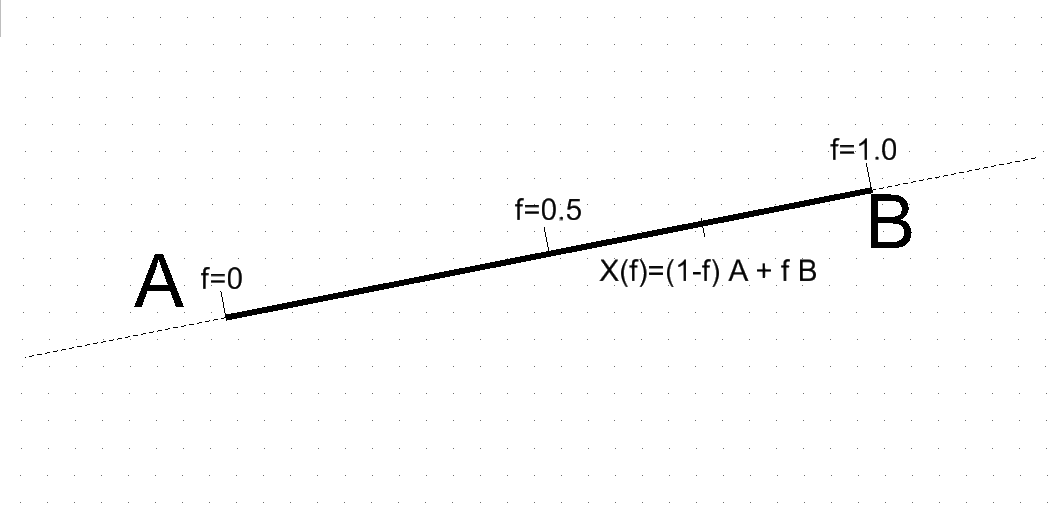• Fractional Parameterization:
    A = start point
B = end point
f = fraction varying from 0 to 1
Point X(f) at fractional position f along the lineSegment is
X(f) = (1-f) * A + f * B

## lineString

• A LineString is an array of points that are to be connected by straight lines.
• Json Fragment: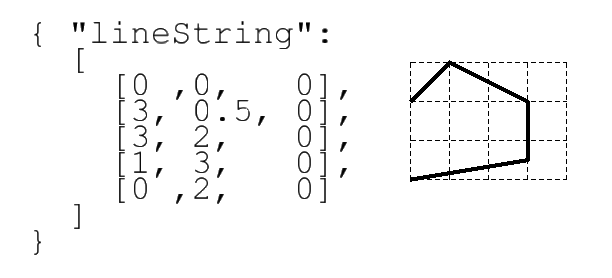• Typescript object:
const myLineString = LineString.create ([point0, point1, point2 ....]);
• Fractional Parameterization

Having both individual line segments and the composite linestring complicates parameterization.

• As with all CurvePrimitives, the fractional parameterization for the complete linestring must have fraction=0 at the start and fraction=1 at the end.
• The fractional positions of each interior vertex are then defined at equal intervals in the fraction space.
•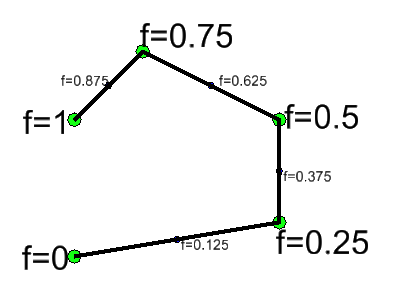• Hence in the example, with 4 segments the vertex fractions increment by one quarter.
• Within each segment, the fraction interval is mapped as if it were a line segment.
• Note that having uniform vertex-to-vertex fraction means that the distance-along-the-linestring is not proportional to fraction-along-entire-linestring. Fraction and distance changes are only proportional within individual segments.

## arcs (circular and elliptic)

An arc primitive is a portion of a circular or elliptical arc. The equations for a complete elliptic arc require a center point and two vectors. The start and end of a partial arc are controlled by two angles.

The equational forms for circular and elliptic cases are identical. Telling whether a given arc is true circular requires examination of the vector coordinates.

The stroking equation that maps an angle to a coordinates to points around a (full) elliptic (or circular) arc is

C = center point
U = vector from center point to 0-degree point
V = vector from center point to 90-degree point.
theta = angle
X(theta) = C + cos (theta * U + sin(theta) * V

### True Circles

• If the U and V vectors are (both) perpendicular and the same length, this is a true circle.
• In the both circles below, the U and V are identical length and perpendicular to each other.
• For the left circle, U and V happen to be in the global x and y directions.
• For the right circle, U and V are still identical length and perpendicular, but are both rotated away from global x and y. This still traces a circle, but the "0 degree" point is moved around the circle.
• When the circular arc conditions are true, the angle used in the equations is an the actual physical angle between the U vector and the vector from the center to X(theta).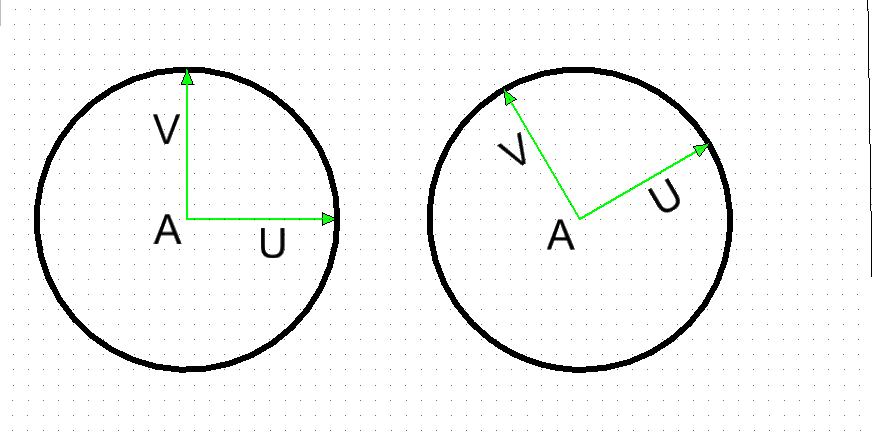### Ellipse

If the U and V vectors either (a) have different lengths or (b) are not perpendicular, the ellipse is non-circular.

If U and V are perpendicular, their lengths correspond to the common usage of "major" and "minor" axis lengths. But the perpendicular condition is not required -- non-perpendicular vectors occur due to transformation and construction history.

### Angular limits

To draw an arc that is not the complete circle or ellipse, simply limit the theta range to something other than 0 to 360 degrees.

theta0 = angular start point
theta1 = angular and point
f = fraction varying from 0 to 1
theta(f) = (1-f) * theta0 + f * theta1
Point X(f) at fractional position f along the arc is

X(f) = C + cos (theta(f)) * U + sin(theta(f)) * V
• Angles theta0 and theta1 can be negative and can be outside of 360 degrees.
• Angle theta1 can be less than theta0

Examples of arc sweep

start and end angles CCW signed sweep angle image
(0 to 360) 360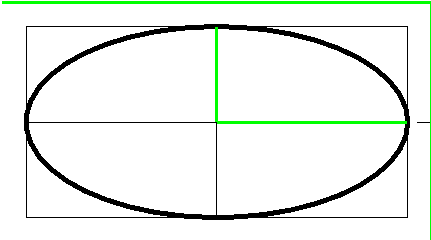(0 to 135) 135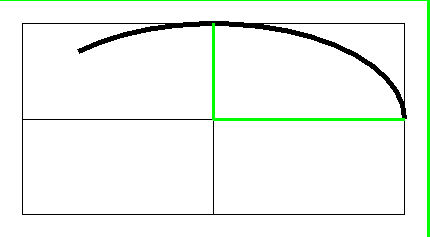(270 to 495) 225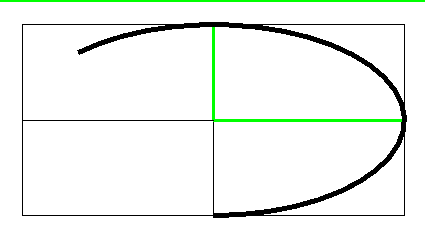(90 to 270) 180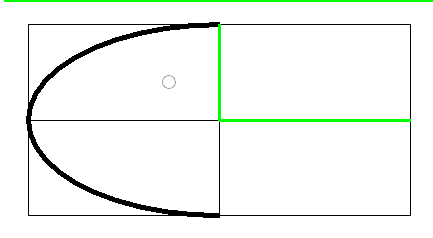(90 to 405) 315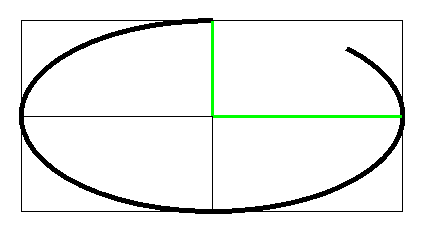Examples with json fragments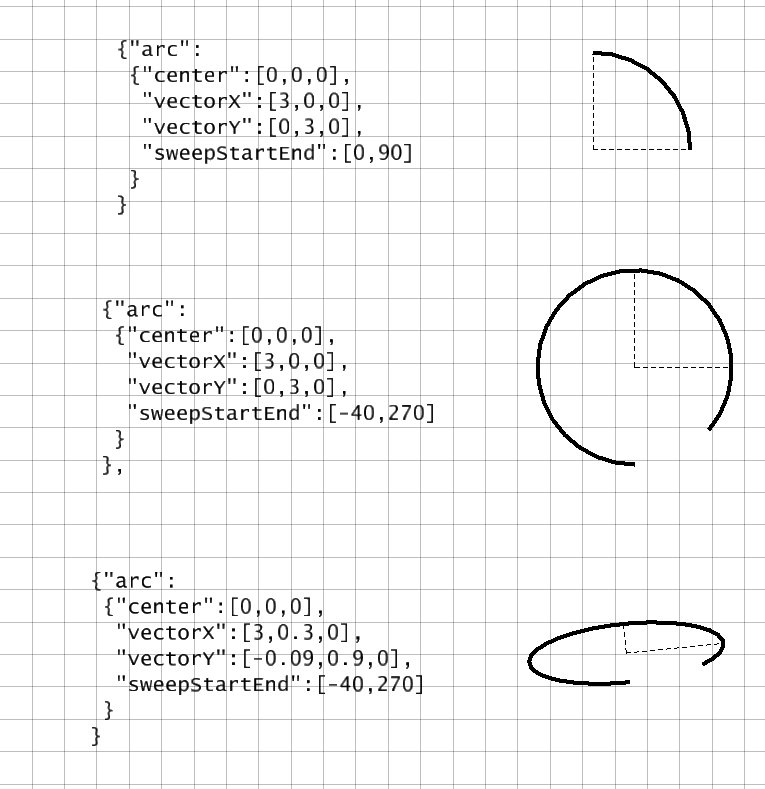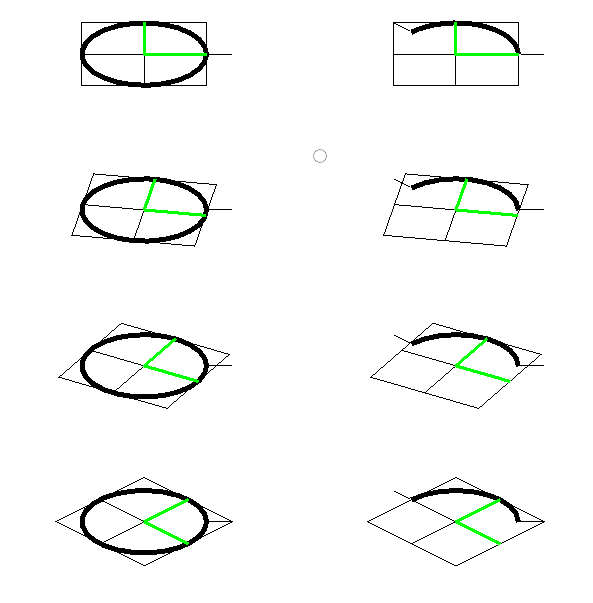# bspline curves

A BSplineCurve3d (or BSplineCurve3dH) is a curve that (loosely) follows a sequence of control points.

Internally, the curve is a is a sequence of polynomial curves that join together smoothly. Call each of those separate pieces a span.

The "control point" structure has remarkable properties for computation:

• The curve never leaves the overall xyz range of the control points.
• This bounding property applies "from any viewpoint", not just in the coordinate system where they are given.
• Even tighter, the curve is contained within the convex hull of the control points.
• No plane can intersect the curve more often than it intersects the control polygon.
• that is, the polygon may overestimate the number of intersections, (i.e. suggest false intersections), but it never underestimates.
• Inspection of the control polygon gives similar "never underestimate" statements about other properties such as
• the number of inflections.
• the number of minima and maxima of the curve and its derivatives.
• The use of "weights" on the control points allows a bspline curve to exactly trace circular and elliptic arcs without use of trig functions.